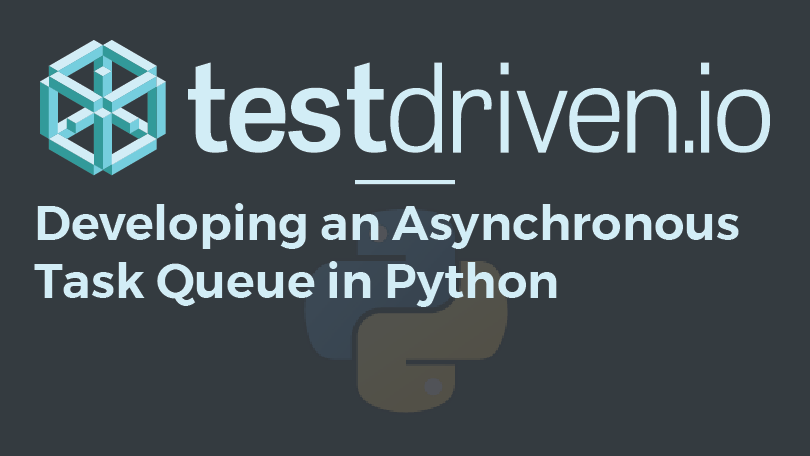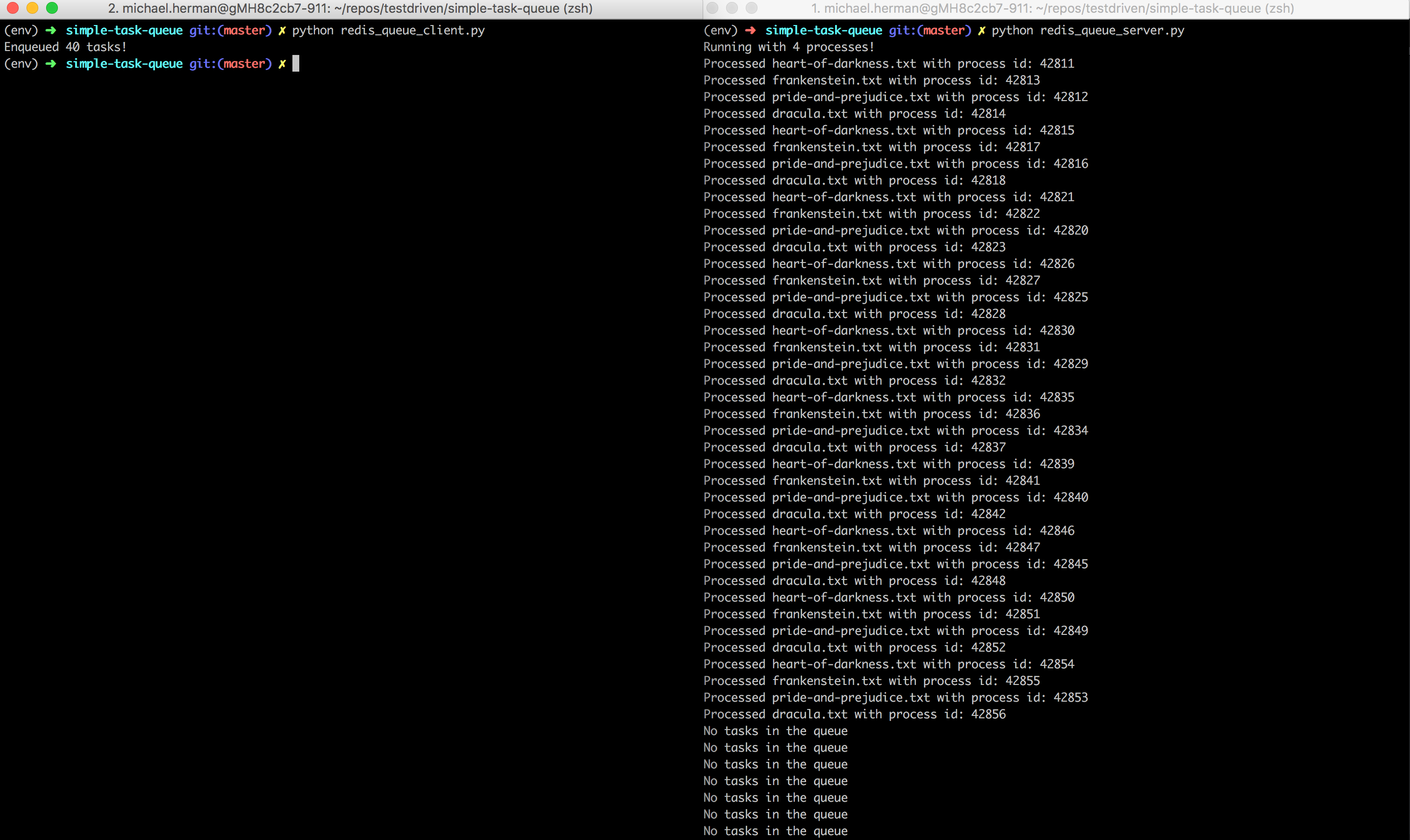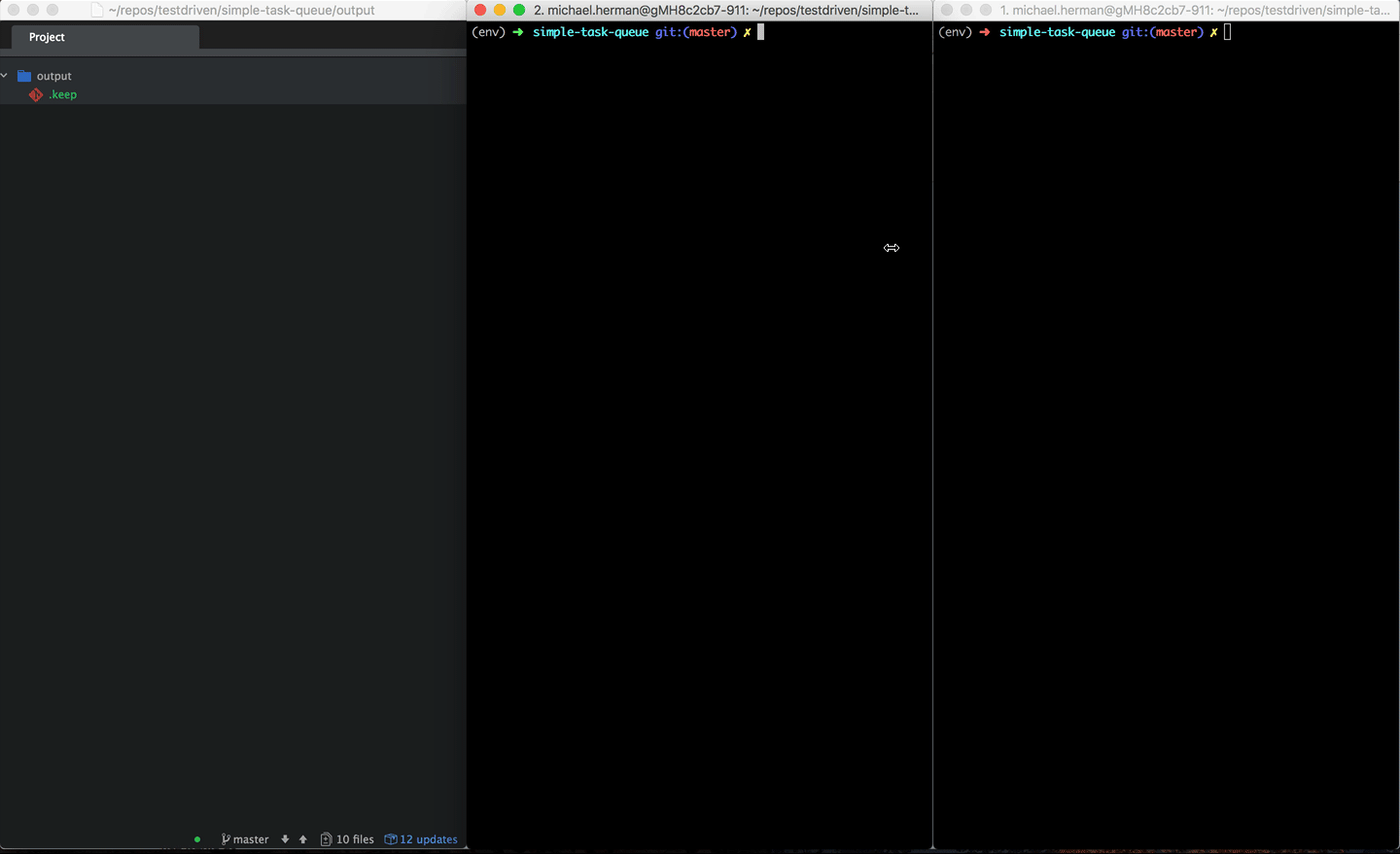## Developing an Asynchronous Task Queue in Python

This post looks at how to implement several asynchronous task queues using Python's multiprocessing library and Redis.

## Queue Data Structures

A queue is a First-In-First-Out (FIFO) data structure.

1. an item is added at the tail (enqueue)
2. an item is removed at the head (dequeue)You'll see this in practice as you code out the examples in this post.

Let's start by creating a basic task:

```# tasks.py

import os
import sys
import json
import time
import uuid
import collections

from nltk.corpus import stopwords

COMMON_WORDS = set(stopwords.words('english'))
DATA_DIRECTORY = os.path.join(os.path.dirname(__file__), 'data')
OUTPUT_DIRECTORY = os.path.join(os.path.dirname(__file__), 'output')

def save_file(filename, data):
random_str = uuid.uuid4().hex
outfile = f'{filename}_{random_str}.txt'
with open(os.path.join(OUTPUT_DIRECTORY, outfile), 'w') as outfile:
outfile.write(data)

def get_word_counts(filename):
wordcount = collections.Counter()
# get counts
with open(os.path.join(DATA_DIRECTORY, filename), 'r') as f:
for line in f:
wordcount.update(line.split())
for word in set(COMMON_WORDS):
del wordcount[word]
# save file
save_file(filename, json.dumps(dict(wordcount.most_common(20))))
time.sleep(2)
proc = os.getpid()
print(f'Processed {filename} with process id: {proc}')

if __name__ == '__main__':
get_word_counts(sys.argv)
```

So, `get_word_counts` finds the twenty most frequent words from a given text file and saves them to an output file. It also prints the current process identifier (or pid) using Python's os library.

#### Following along?

Create a project directory along with a virtual environment. Then, use pip to install NLTK:

```\$ pip install nltk==3.4.1
```

Once installed, invoke the Python shell and download the `stopwords` corpus:

```>>> import nltk
[nltk_data]     /Users/michael.herman/nltk_data...
[nltk_data]   Unzipping corpora/stopwords.zip.
True
```

Example fix:

```>>> import nltk
[nltk_data]     CERTIFICATE_VERIFY_FAILED] certificate verify failed:
[nltk_data]     unable to get local issuer certificate (_ssl.c:1056)>
False
>>> import ssl
>>> try:
...     _create_unverified_https_context = ssl._create_unverified_context
... except AttributeError:
...     pass
... else:
...     ssl._create_default_https_context = _create_unverified_https_context
...
[nltk_data]     /Users/michael.herman/nltk_data...
[nltk_data]   Unzipping corpora/stopwords.zip.
True
```

## Multiprocessing Pool

We can run this task in parallel using the multiprocessing library:

```# simple_pool.py

import time
import multiprocessing

PROCESSES = multiprocessing.cpu_count() - 1

def run():
print(f'Running with {PROCESSES} processes!')
start = time.time()
with multiprocessing.Pool(PROCESSES) as p:
p.map_async(get_word_counts, [
'pride-and-prejudice.txt',
'heart-of-darkness.txt',
'frankenstein.txt',
'dracula.txt'
])
# clean up
p.close()
p.join()
print(f'Time taken = {time.time() - start:.10f}')

if __name__ == '__main__':
run()
```

Here, using the Pool class, we processed four tasks with two processes.

Did you notice the `map_async` method? There are essentially four different methods available for mapping tasks to processes. When choosing one, you have to take multi-args, concurrency, blocking, and ordering into account:

Method Multi-args Concurrency Blocking Ordered-results
`map` No Yes Yes Yes
`map_async` No No No Yes
`apply` Yes No Yes No
`apply_async` Yes Yes No No

Without both `close` and `join`, garbage collection may not occur, which could lead to a memory leak.

1. `close` tells the pool not to accept any new tasks
2. `join` tells the pool to exit after all tasks have completed

Following along? Grab the Project Gutenberg sample text files from the "data" directory in the simple-task-queue repo, and then add an "output" directory.

Your project directory should look like this:

```├── data
│   ├── dracula.txt
│   ├── frankenstein.txt
│   ├── heart-of-darkness.txt
│   └── pride-and-prejudice.txt
├── output
├── simple_pool.py
```

It should take about two seconds to run:

```(env)\$ python simple_pool.py

Running with 7 processes!
Processed heart-of-darkness.txt with process id: 27713
Processed frankenstein.txt with process id: 27714
Processed pride-and-prejudice.txt with process id: 27712
Processed dracula.txt with process id: 27715
Time taken = 2.1577479839
```

This script ran on a i7 Macbook Pro with 8 cores.

So, the multiprocessing `Pool` class handles the queuing logic for us. It's perfect for running web scraping jobs in parallel (example) or really any job that can be broken up and distributed independently. If you need more control over the queue or need to share data between multiple processes, you may want to look at the `Queue` class.

## Multiprocessing Queue

Let's look at a simple example:

```# simple_queue.py

import multiprocessing

def run():
books = [
'pride-and-prejudice.txt',
'heart-of-darkness.txt',
'frankenstein.txt',
'dracula.txt'
]
queue = multiprocessing.Queue()

print('Enqueuing...')
for book in books:
print(book)
queue.put(book)

print('\nDequeuing...')
while not queue.empty():
print(queue.get())

if __name__ == '__main__':
run()
```

The Queue class, also from the multiprocessing library, is a basic FIFO (first in, first out) data structure. It's similar to the queue.Queue class, but designed for interprocess communication. We used `put` to enqueue an item to the queue and `get` to dequeue an item.

Check out the `Queue` source code for a better understanding of the mechanics of this class.

Now, let's look at more advanced example:

```# simple_task_queue.py

import time
import multiprocessing

PROCESSES = multiprocessing.cpu_count() - 1

get_word_counts(book)
return True

def run():
processes = []
print(f'Running with {PROCESSES} processes!')
start = time.time()
for n in range(PROCESSES):
p = multiprocessing.Process(
processes.append(p)
p.start()
for p in processes:
p.join()
print(f'Time taken = {time.time() - start:.10f}')

if __name__ == '__main__':
run()
```

Here, we enqueued 40 tasks (ten for each text file) to the queue, created separate processes via the `Process` class, used `start` to start running the processes, and, finally, used `join` to complete the processes.

It should take about 12 seconds to run.

Challenge: Check your understanding by adding another queue to hold completed tasks. You can enqueue them within the `process_tasks` function.

## Logging

The multiprocessing library provides support for logging as well:

```# simple_task_queue_logging.py

import os
import time
import logging
import multiprocessing

PROCESSES = multiprocessing.cpu_count() - 1

logger = multiprocessing.get_logger()
proc = os.getpid()
try:
get_word_counts(book)
except Exception as e:
logger.error(e)
logger.info(f'Process {proc} completed successfully')
return True

def run():
processes = []
print(f'Running with {PROCESSES} processes!')
start = time.time()
for w in range(PROCESSES):
p = multiprocessing.Process(
processes.append(p)
p.start()
for p in processes:
p.join()
print(f'Time taken = {time.time() - start:.10f}')

if __name__ == '__main__':
multiprocessing.log_to_stderr(logging.ERROR)
run()
```

To test, change `task_queue.put('dracula.txt')` to `task_queue.put('drakula.txt')`. You should see the following error outputted ten times in the terminal:

```[ERROR/Process-4] [Errno 2] No such file or directory:
```

Want to log to disc?

```# simple_task_queue_logging.py

import os
import time
import logging
import multiprocessing

PROCESSES = multiprocessing.cpu_count() - 1

def create_logger():
logger = multiprocessing.get_logger()
logger.setLevel(logging.INFO)
fh = logging.FileHandler('process.log')
fmt = '%(asctime)s - %(levelname)s - %(message)s'
formatter = logging.Formatter(fmt)
fh.setFormatter(formatter)
return logger

logger = create_logger()
proc = os.getpid()
try:
get_word_counts(book)
except Exception as e:
logger.error(e)
logger.info(f'Process {proc} completed successfully')
return True

def run():
processes = []
print(f'Running with {PROCESSES} processes!')
start = time.time()
for w in range(PROCESSES):
p = multiprocessing.Process(
processes.append(p)
p.start()
for p in processes:
p.join()
print(f'Time taken = {time.time() - start:.10f}')

if __name__ == '__main__':
run()
```

Again, cause an error by altering one of the file names, and then run it. Take a look at process.log. It's not quite as organized as it should be since the Python logging library does not use shared locks between processes. To get around this, let's have each process write to its own file. To keep things organized, add a logs directory to your project folder:

```# simple_task_queue_logging_separate_files.py

import os
import time
import logging
import multiprocessing

PROCESSES = multiprocessing.cpu_count() - 1

def create_logger(pid):
logger = multiprocessing.get_logger()
logger.setLevel(logging.INFO)
fh = logging.FileHandler(f'logs/process_{pid}.log')
fmt = '%(asctime)s - %(levelname)s - %(message)s'
formatter = logging.Formatter(fmt)
fh.setFormatter(formatter)
return logger

proc = os.getpid()
logger = create_logger(proc)
try:
get_word_counts(book)
except Exception as e:
logger.error(e)
logger.info(f'Process {proc} completed successfully')
return True

def run():
processes = []
print(f'Running with {PROCESSES} processes!')
start = time.time()
for w in range(PROCESSES):
p = multiprocessing.Process(
processes.append(p)
p.start()
for p in processes:
p.join()
print(f'Time taken = {time.time() - start:.10f}')

if __name__ == '__main__':
run()
```

## Redis

Moving right along, instead of using an in-memory queue, let's add Redis into the mix.

Following along? Download and install Redis if you do not already have it installed. Then, install the Python interface:

```\$ pip install redis==3.2.1
```

We'll break the logic up into four files:

1. redis_queue.py creates new queues and tasks via the `SimpleQueue` and `SimpleTask` classes, respectively.
3. redis_queue_worker dequeues and processes tasks.
4. redis_queue_server spawns worker processes.
```# redis_queue.py

import uuid
import pickle

class SimpleQueue(object):
def __init__(self, conn, name):
self.conn = conn
self.name = name

def enqueue(self, func, *args):

def dequeue(self):

def get_length(self):
return self.conn.llen(self.name)

def __init__(self, func, *args):
self.id = str(uuid.uuid4())
self.func = func
self.args = args

self.func(*self.args)
```

Here, we defined two classes, `SimpleQueue` and `SimpleTask`:

1. `SimpleQueue` creates a new queue and enqueues, dequeues, and gets the length of the queue.
2. `SimpleTask` creates new tasks, which are used by the instance of the `SimpleQueue` class to enqueue new tasks, and processes new tasks.

Curious about `lpush()`, `brpop()`, and `llen()`? Refer to the Command reference page. (`The brpop()` function is particularly cool because it blocks the connection until a value exists to be popped!)

```# redis_queue_client.py

import redis

from redis_queue import SimpleQueue

if __name__ == '__main__':
r = redis.Redis()
queue = SimpleQueue(r, 'sample')
count = 0
queue.enqueue(get_word_counts, 'pride-and-prejudice.txt')
queue.enqueue(get_word_counts, 'heart-of-darkness.txt')
queue.enqueue(get_word_counts, 'frankenstein.txt')
queue.enqueue(get_word_counts, 'dracula.txt')
count += 4
```

This module will create a new instance of Redis and the `SimpleQueue` class and enqueue 40 tasks.

```# redis_queue_worker.py

import redis

from redis_queue import SimpleQueue

def worker():
r = redis.Redis()
queue = SimpleQueue(r, 'sample')
if queue.get_length() > 0:
queue.dequeue()
else:

if __name__ == '__main__':
worker()
```

If there is an available task, the `dequeue` method is called, which then de-serializes the task and calls the `process_task` method (in redis_queue.py).

```# redis_queue_server.py

import os
import logging
import multiprocessing

from redis_queue_worker import worker

PROCESSES = 4

def run():
processes = []
print(f'Running with {PROCESSES} processes!')
while True:
for w in range(PROCESSES):
p = multiprocessing.Process(target=worker)
processes.append(p)
p.start()
for p in processes:
p.join()

if __name__ == '__main__':
run()
```

The `run` method spawns four new worker processes.

You probably don’t want four processes running at once all the time, but there may be times that you will need four or more processes. Think about how you could programmatically spin up and down additional workers based on demand.

To test, run redis_queue_server.py and redis_queue_client.py in separate terminal windows:## Conclusion

In this post, we looked at a number of asynchronous task queue implementations in Python. If the requirements are simple enough, it may be easier to develop a queue in this manner. That said, if you're looking for more advanced features -- like task scheduling, batch processing, job prioritization, and retrying of failed tasks -- you should look into a full-blown solution. Check out Celery, RQ, or Huey.

Grab the final code from the simple-task-queue repo.